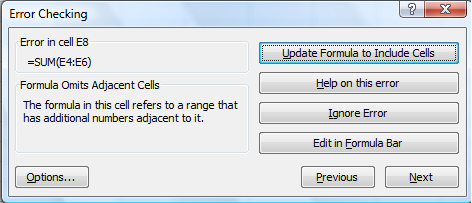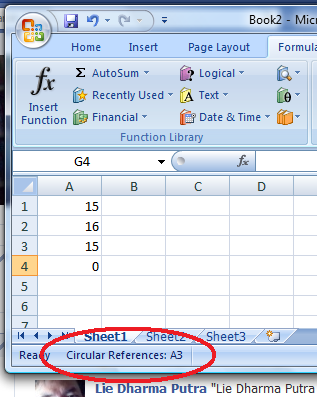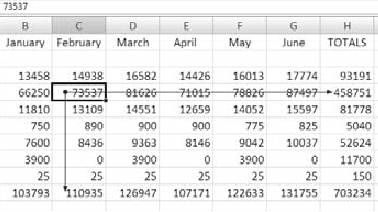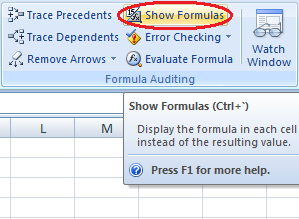Connect with us

# 4 Steps To Troubleshoot Formula Errors in Excel 2007Published

onIn general, accountant known as the most sophisticated excel user. But, as other aspect of our life, even when we performed things correctly, there is no guarantee that everything is correct as what we expect to be. Shit can happened. As with excel formula, even when a formula appears to be entered correctly, the order of the functions or operations may not be correct in order to get the results you want. In this case, we need to trouble shoot the formula which shows errors. To trouble shoot error formula in excel, there are four basic steps you can take as I discuss in this post.

Step-1. Choose Formulas => Formula Auditing => Error Checking.

If Excel finds potential formula errors in your worksheet, it jumps to the cell containing the error and displays the Error Checking dialog box shown below:If the worksheet contains no errors, Excel displays a message box saying the error-checking is complete.

Here are just a few of the error types Excel might locate:

DIV/0!: Divide by zero error. This error means the formula is trying to divide by either an empty cell or one with a value of zero. Make sure all cells referenced in the division have a value other than zero in them.

#VALUE!: This means the formula references an invalid cell address. For example, text is in a cell where the formula is expecting to find a value. You might also see this error if you delete a value in a cell that was used in a formula. Locate and correct the invalid cell reference.

NAME#: This occurs when Excel doesn’t recognize text in a formula, perhaps because of a misspelling of a range name. Make sure the name actually exists and is spelled correctly. Also verify the spelling of the function name.

Circular: This means that the formula in a cell is referring to itself. Locate the circular reference and edit the formula so it does not include itself. Figure 3-16 shows a circular reference.Number Stored as Text: This means a cell contains what looks like a value, but the content is stored as text. Verify that you did intend to store the value as a text entry.

Formula Omits Adjacent Cells: This means a formula refers to a group of cells that have numbers adjacent to it. Study the formula to make sure you didn’t forget to include additional cell references in the formula.

Step-2. Perform one of the following steps, depending on the error type:

• Click in the cell and edit the formula as needed. Click Resume when you are finished.
• Click an option, if available, to allow the Error Checking dialog box to assist you with repairing the problem.

Step-3. Click Next to review the next problem area. When the error-check process is complete, Excel displays a message box.

Step-4. Click OK.

### Identify Formula Precedents and Dependents

Step-1. Select a cell that contains a formula or one that is referenced in one or more formulas.

Step-2. Choose Formulas => Formula Auditing => Trace Precedents or Trace Dependents. Excel displays one or more blue arrows pointing out either precedents or, as shown below:Step-3. Click Remove Arrows. The blue arrows disappear.

To help you determine the nature or origin of the problem, some error messages have a Show Calculation Steps button to display the logic behind the formula.

Another great troubleshooting tool is the capability to display the actual formula, instead of the result, in the worksheet. Choose Formulas?Formula Auditing?Show Formulas. Click the same button again to return to the formula result.Dependent cells are those that are referred to by a formula in another cell. Precedent cells are those that contain formulas that refer to other cells.

Are you looking for easy accounting tutorial? Established since 2007, Accounting-Financial-Tax.com hosts more than 1300 articles (still growing), and has helped millions accounting student, teacher, junior accountants and small business owners, worldwide.